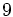# Groups of order 512

## Contents

Note that since$512 = 2^9$ is a prime power, and prime power order implies nilpotent, all groups of order 512 are nilpotent groups.
Number of abelian groups up to isomorphism 30 4 Equals the number of unordered integer partitions of$9$. See classification of finite abelian groups.
Number of maximal class groups, i.e., groups of nilpotency class exactly$9 - 1 = 8$ 3 1 The dihedral group, semidihedral group, and generalized quaternion group. See classification of finite 2-groups of maximal class.